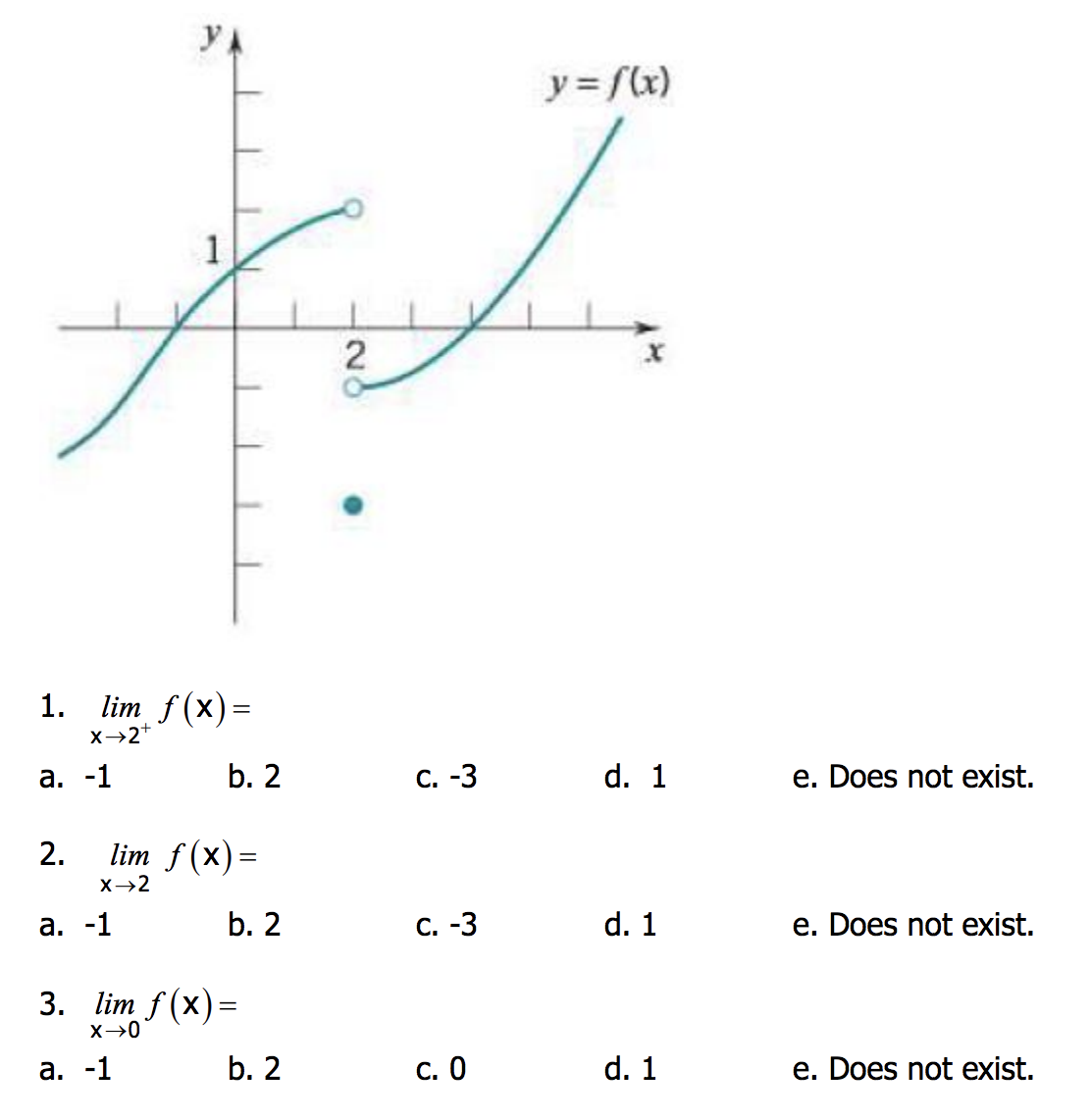# 2 1. lim f(x)- x-2+ a. b. 2 C. d. 1 e. Does not exist. 2. lin2 f(x)= a. b. 2 C. d. 1 e. Does not exist. 3. lim f(x)- x→0 a. b. 2 c. O d. 1 e. Does not exist.

Question

How do i find these limits? For example for number1, since there are 3 points at 2 would it be DNE? or since its coming from the right would it be -1 and i ignore the other point and curve?help_outlineImage Transcriptionclose2 1. lim f(x)- x-2+ a. b. 2 C. d. 1 e. Does not exist. 2. lin2 f(x)= a. b. 2 C. d. 1 e. Does not exist. 3. lim f(x)- x→0 a. b. 2 c. O d. 1 e. Does not exist. fullscreen

### Want to see this answer and more?

Experts are waiting 24/7 to provide step-by-step solutions in as fast as 30 minutes!*